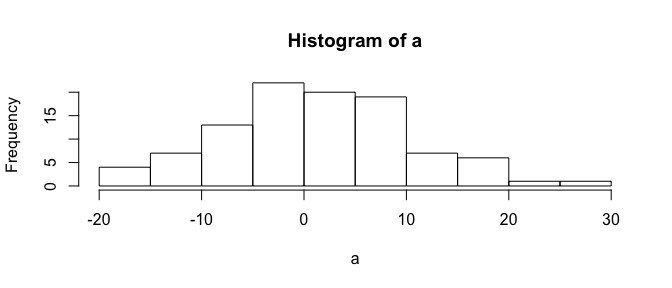# 1 First Steps

This tutorial has been forked from awesome classes developed by Adam Wilson here: http://adamwilson.us/RDataScience/

The R Script associated with this page is available here. Download this file and open it (or copy-paste into a new script) with RStudio so you can follow along.

## 1.1 Variables

``````x=1
x``````
``##  1``

We can also assign a vector to a variable:

``````x=c(5,8,14,91,3,36,14,30)
x``````
``##   5  8 14 91  3 36 14 30``

And do simple arithmetic:

``x+2``
``##   7 10 16 93  5 38 16 32``

Create a new variable called `y` and set it to `15`

``y=15``

Note that `R` is case sensitive, if you ask for `X` instead of `x`, you will get an error

``````X

### 1.1.1 Variable naming conventions

• alllowercase: e.g. `adjustcolor`
• period.separated: e.g. `plot.new`
• underscore_separated: e.g. `numeric_version`
• lowerCamelCase: e.g. `addTaskCallback`
• UpperCamelCase: e.g. `SignatureMethod`

# 2 Subsetting

``x``
``##   5  8 14 91  3 36 14 30``

Subset the vector using `x[ ]` notation

``x``
``##  3``

You can use a `:` to quickly generate a sequence:

``1:5``
``##  1 2 3 4 5``

and use that to subset as well:

``x[1:5]``
``##   5  8 14 91  3``

# 3 Using Functions

To calculate the mean, you could do it manually like this

``(5+8+14+91+3+36+14+30)/8``
``##  25.125``

Or use a function:

``mean(x)``
``##  25.125``

Type `?functionname` to get the documentation (`?mean`) or `??"search parameters` (??“standard deviation”) to search the documentation. In RStudio, you can also search in the help panel. `mean` has other arguments too:

`mean(x, trim = 0, na.rm = FALSE, ...)`

In RStudio, if you press `TAB` after a function name (such as `mean(`), it will show function arguments.Autocomplete screenshot

Calculate the standard deviation of `c(3,6,12,89)`.

``````y=c(3,6,12,89)
sqrt((sum((y-mean(y))^2))/(length(y)-1))``````
``##  41.17038``
``````#or
sd(y)``````
``##  41.17038``
``````#or
sd(c(3,6,12,89))``````
``##  41.17038``

Writing functions in R is pretty easy. Let’s create one to calculate the mean of a vector by getting the sum and length. First think about how to break it down into parts:

``````x1= sum(x)
x2=length(x)
x1/x2``````
``##  25.125``

Then put it all back together and create a new function called `mymean`:

``````mymean=function(f){
sum(f)/length(f)
}

mymean(f=x)``````
``##  25.125``

Confirm it works:

``mean(x)``
``##  25.125``
Any potential problems with the `mymean` function?

# 4 Missing data: dealing with `NA` values

``x3=c(5,8,NA,91,3,NA,14,30,100)``
" What do you think `mymean(x3)` will return?

Calculate the mean using the new function

``mymean(x3)``
``##  NA``

Use the built-in function (with and without na.rm=T)

``mean(x3)``
``##  NA``
``mean(x3,na.rm=T)``
``##  35.85714``

Writing simple functions is easy, writing robust, reliable functions can be hard…

## 4.1 Logical values

R also has standard conditional tests to generate `TRUE` or `FALSE` values (which also behave as `0`s and `1`s. These are often useful for filtering data (e.g. identify all values greater than 5). The logical operators are `<`, `<=`, `>`, `>=`, `==` for exact equality and `!=` for inequality.

``  x``
``##   5  8 14 91  3 36 14 30``
``  x3 > 75``
``##  FALSE FALSE    NA  TRUE FALSE    NA FALSE FALSE  TRUE``
``  x3 == 40``
``##  FALSE FALSE    NA FALSE FALSE    NA FALSE FALSE FALSE``
``  x3 >   15``
``##  FALSE FALSE    NA  TRUE FALSE    NA FALSE  TRUE  TRUE``

And you can perform operations on those results:

``sum(x3>15,na.rm=T)``
``##  3``

or save the results as variables:

``````result =  x3 >  3
result``````
``##   TRUE  TRUE    NA  TRUE FALSE    NA  TRUE  TRUE  TRUE``

Define a function that counts how many values in a vector are less than or equal (`<=`) to 12.

``````mycount=function(x){
sum(x<=12)
}``````

Try it:

``x3``
``##    5   8  NA  91   3  NA  14  30 100``
``mycount(x3)``
``##  NA``

oops!

``````mycount=function(x){
sum(x<=12,na.rm=T)
}``````

Try it:

``x3``
``##    5   8  NA  91   3  NA  14  30 100``
``mycount(x3)``
``##  3``

Nice!

# 5 Generating Data

There are many ways to generate data in R such as sequences:

``seq(from=0, to=1, by=0.25)``
``##  0.00 0.25 0.50 0.75 1.00``

and random numbers that follow a statistical distribution (such as the normal):

``a=rnorm(100,mean=0,sd=10)``

Let’s visualize those values in a histogram:

``hist(a)``We’ll cover much more sophisticated graphics later…

# 6 Data Types

## 6.1 Matrices

You can also use matrices (2-dimensional arrays of numbers):

``````y=matrix(1:9,ncol=3)
y``````
``````##      [,1] [,2] [,3]
## [1,]    1    4    7
## [2,]    2    5    8
## [3,]    3    6    9``````

Matrices behave much like vectors:

``y+2``
``````##      [,1] [,2] [,3]
## [1,]    3    6    9
## [2,]    4    7   10
## [3,]    5    8   11``````

and have 2-dimensional indexing:

``y[2,3]``
``##  8``

Create a 3x3 matrix full of random numbers. Hint: `rnorm(5)` will generate 5 random numbers

``matrix(rnorm(9),nrow=3)``
``````##            [,1]       [,2]      [,3]
## [1,] -0.6385807 -1.1242050 0.1428754
## [2,] -0.6640297 -0.4913995 0.3565074
## [3,] -0.5751638  0.1669154 0.5599687``````

## 6.2 Data Frames

Data frames are similar to matrices, but more flexible. Matrices must be all the same type (e.g. all numbers), while a data frame can include multiple data types (e.g. text, factors, numbers). Dataframes are commonly used when doing statistical modeling in R.

``````data = data.frame( x = c(11,12,14),
y = c("a","b","b"),
z = c(T,F,T))
data``````
``````##    x y     z
## 1 11 a  TRUE
## 2 12 b FALSE
## 3 14 b  TRUE``````

You can subset in several ways

``mean(data\$x)``
``##  12.33333``
``mean(data[["x"]])``
``##  12.33333``
``mean(data[,1])``
``##  12.33333``

For installed packages: `library(packagename)`.
New packages: `install.packages()` or use the package manager.
``library(raster)``
If you don’t have the packages above, install them in the package manager or by running `install.packages("raster")`.